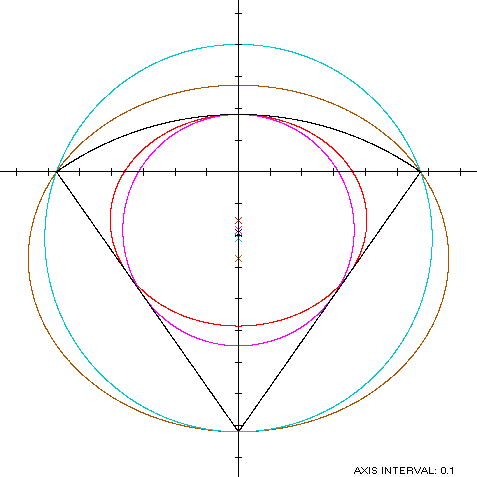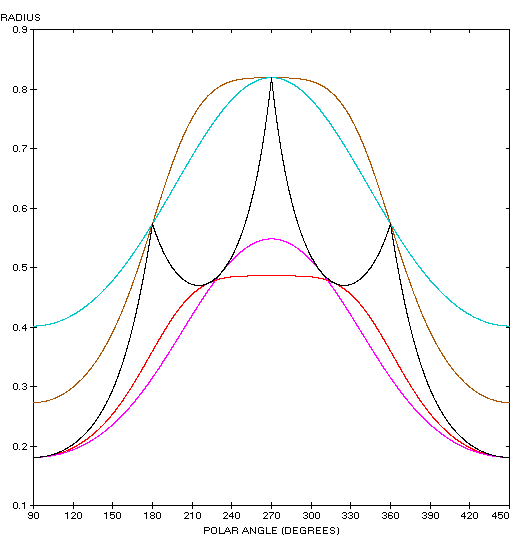Circular Sector
CIRCULAR SECTOR

Balmoral Software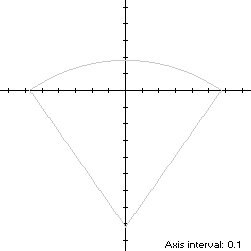Let a circular sector S of radius 1 be oriented with the origin at the midpoint of the chord connecting the endpoints of its arc, and with the center of the circle on the negative y-axis, so that S is symmetric with respect to that axis. If β < π/2 is one-half the angle subtended by the sector arc, then the extreme abscissa points of S are (±sin(β),0), the minimum ordinate point is (0,-cos(β)), the maximum ordinate point is (0,1 - cos(β)), and the width x height of the bounding rectangle of S is 2sin(β) x 1.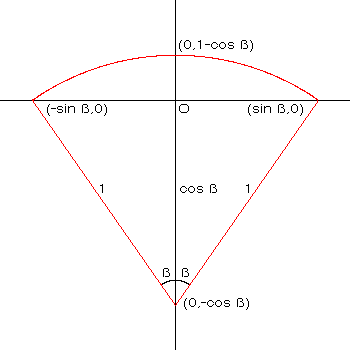Let t represent the usual parameter, ranging from 0 to 2π through the quadrants in counterclockwise order. In Quadrants I and II, the parametric equations of the sector arc are:
x(φ) = cos(φ)

y(φ) = sin(φ) - cos(β),

where the angle φ is measured from the center of the circle and ranges from π/2 - β to π/2 + β. We can scale t to the angular parameter φ as follows:
 Range of t Range of φ [0,π] [π/2 - β,π/2 + β]
or equivalently,
φ = 2βt/π + π/2 - β
In Quadrants III and IV, S consists of symmetric line segments joining the points (-sin(β),0), (0,-cos(β)) and (sin(β),0), so the parametric functions are linear in t. Combining definitions, we have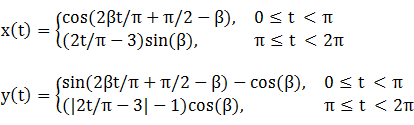### Metrics

The perimeter of the sector is the length of the circular arc (in radians) plus its two radii:
L = 2β + 2
The area A of S is β radians and its centroid ordinate is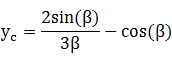Specific Example

In the remainder of this paper, we use

β = 35° = 7π/36

L = 3.221731
A = 0.610865
d = -0.193180
Bounding rectangle: 1.147153 x 1

### Incircle

Using z = 1 - cos(β) in Lemma C, we have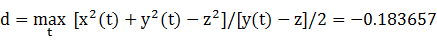R = |d - z| = 0.364505

For verification, we have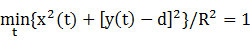### Inellipse

Using z = 1 - cos(β) in Lemma E,
d/dt x(t)[y(t) - z] = d/dt (2t/π - 3)sin(β)[|2t/π - 3|cos(β) - 1]
has a zero in [π,2π) when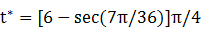The corresponding coordinates are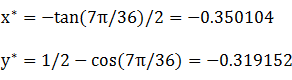We then have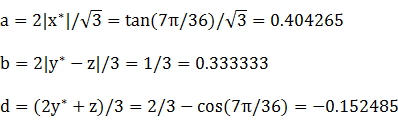For verification,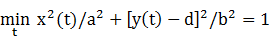### Circumcircle

A circumcircle passing through the three corners of S will have a smaller radius than that of the sector itself, and so will have a greater curvature and contain the circular arc portion of S. Applying Lemma TC to an inverted acute isosceles triangle with h = cos(β) and w = 2sin(β), the center ordinate (negated) and the radius of the circumcircle are:
d = h/2 - w2/(8h) = cos(2β)sec(β)/2 = -0.208765

R = h - d = sec(β)/2 = 0.610387

### Circumellipse

Using the same approach as for the circumcircle, we apply Lemma TE with h = cos(β) and w = 2sin(β) to find the parameters of the circumellipse: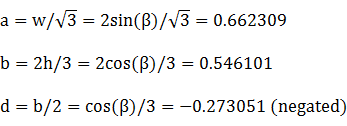### Summary Table

 Perimeter Area Centroid Figure Parameters Incircle R = 0.364505 2.290253 0.417404 (0,-0.183657) Inellipse a = tan(7π/36)/\/3b = 1/3 2.322593 0.423345 (0,-0.152485) Circular sector Width: 2sin(β)Height: 1 3.221731 0.610865 (0,-0.193180) Circumellipse a =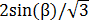b =3.805114 1.136275 (0,-0.273051) Circumcircle R = sec(β)/2 3.835177 1.170472 (0,-0.208765)